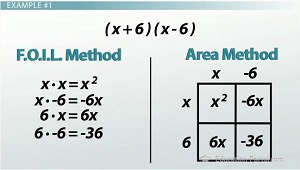# Multiplying Binomials Using FOIL & the Area Method: Practice Problems

An error occurred trying to load this video.

Try refreshing the page, or contact customer support.

Coming up next: How to Factor Quadratic Equations: FOIL in Reverse

### You're on a roll. Keep up the good work!

Replay
Your next lesson will play in 10 seconds
• 0:05 Mutiplying Binomials
• 0:45 Example #1
• 2:28 Example #2
• 3:44 Example #3
• 4:48 Area Method and Other…
• 5:08 Lesson Summary

Want to watch this again later?

Timeline
Autoplay
Autoplay
Speed

#### Recommended Lessons and Courses for You

Lesson Transcript
Instructor: Luke Winspur

Luke has taught high school algebra and geometry, college calculus, and has a master's degree in education.

There are a few mistakes that are easy to make when multiplying binomials with FOIL and also a few ways to complicate problems like this, so why not make sure you're brushed up on your skills? You'll also learn a shortcut and how to use the area method to multiply even bigger polynomials.

## Multiplying Binomials

Kind of like learning your times tables, multiplying binomials is one of those skills that can start out slow but with some practice become really fast and easy. This lesson is here to help you move from slow and unsure to fast and confident!

Because there are so many different ways to multiply two binomials, we'll do each problem in this lesson two ways. I won't explain both ways word-for-word in each problem, but we'll have both ways going on the screen at once so you can pick which of them you like more and also see how they're basically the same thing. Feel free to pause the lesson when I introduce a problem and give it a shot on your own before you watch me do it. That way you can either focus on the specific mistake you make or quickly skip through the problem if you got it right!

## Example #1

Let's start with a pretty basic one.

Multiply (x + 6)(x - 6)

We'll use the FOIL method on the left side of the screen and the area method on the right. Remember that both are simply procedures that help you remember the four mini-multiplication problems that these examples will break down into. FOIL uses an acronym to help you remember which things to multiply, while the area method uses a chart to do the same thing. FOIL is a little bit quicker but also easier to make mistakes with, while the area method takes a little bit more time because you need to draw out the chart, but it's much better at preventing silly mistakes.

Because the numbers aren't too bad on this one, I'll go ahead and use FOIL. That means I begin by multiplying the First terms, x and x, to get x2.

Then we do the Outer terms, x and -6, to get -6x.

The Inner terms, 6 and x, give us 6x.

And finally we have the Last terms, 6 and -6, leaving us with a -36 on the end.

x2 - 6x + 6x - 36We're already basically done, but we'd like to simplify our answer by combining like terms. I've got two groups of xs here, and when we do -6x + 6x, they will actually cancel each other out, leaving our answer as simply:

x2 - 36

Now, there is actually a shortcut to answering problems like this where you are multiplying two conjugates together. Two binomials are conjugates if they have the same two terms but opposite signs on the second one like (a + b) and (a - b). Any time we multiply two conjugates together, the two middle terms will drop out, just like we saw, giving us the shortcut:

(a + b)(a - b) = a2 - b2

## Example #2

Let's move on to another example:

Multiply (2x + 5)2

I bring this example up for one important reason: it's good to learn that this problem should be done like the other ones in this video, even though it doesn't look like it. If you're not aware of this, it can be very easy to make this simple mistake:

2x2 + 52

Distributing the exponent like this is not allowed! Here's the reason. Squaring something means multiplying it by itself. That means taking 2x + 5 and multiplying it by 2x + 5. Aha! Two binomials! If you make the mistake of distributing the exponent, you are only doing the first and last steps of FOIL and skipping the middle two - not good!

To unlock this lesson you must be a Study.com Member.

### Register to view this lesson

Are you a student or a teacher?

### Unlock Your Education

#### See for yourself why 30 million people use Study.com

##### Become a Study.com member and start learning now.
Back
What teachers are saying about Study.com

### Earning College Credit

Did you know… We have over 160 college courses that prepare you to earn credit by exam that is accepted by over 1,500 colleges and universities. You can test out of the first two years of college and save thousands off your degree. Anyone can earn credit-by-exam regardless of age or education level.